Processing ......FreeComputerBooks.com Links to Free Computer, Mathematics, Technical Books all over the World

Discrete Structures, Logic, and Computability
Top Free C Programming Books 🌠 - 100% Free or Open Source!
• Title: Discrete Structures, Logic, and Computability
• Author(s) James L. Hein
• Publisher: Jones & Bartlett Publishers; 3 edition (February 26, 2009)
• Paperback: N/A
• eBook: PDF (161 pages, 4.3 MB)
• Language: English
• ISBN-10: 0763772062
• ISBN-13: 978-0763772062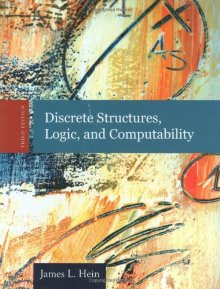Book Description

Thoroughly updated, the new Third Edition of Discrete Structures, Logic, and Computability introduces beginning computer science and computer engineering students to the fundamental techniques and ideas used by computer scientists today, focusing on topics from the fields of mathematics, logic, and computer science itself.

Dr. Hein provides elementary introductions to those ideas and techniques that are necessary to understand and practice the art and science of computing. The text contains all the topics for discrete structures in the reports of the IEEE/ACM Joint Task Force on Computing Curricula for computer science programs and for computer engineering programs.

• N/A

Reviews, Ratings, and Recommendations: Related Book Categories: Read and Download Links:Similar Books:
•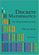Discrete Mathematics: An Open Introduction (Oscar Levin)

This is a gentle introduction to discrete mathematics. Four main topics are covered: counting, sequences, logic, and graph theory. Along the way proofs are introduced, including proofs by contradiction, proofs by induction, and combinatorial proofs.

•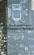Applied Discrete Structures, Fundamentals (Al Doerr, et al)

This book contains most of the fundamental concepts taught in a one semester course in discrete mathematics which is a required course for students in Computer Science, Mathematics and Information Technology.

•Discrete Structures for Computer Science: Counting, Recursion

This book provides a broad introduction to some of the most fascinating and beautiful areas of discrete mathematical structures with examples in logic, applications of the principle of inclusion and exclusion and finally the pigeonhole principal.

•Mathematics for Computer Science (Eric Lehman, et al)

This book covers elementary discrete mathematics for computer science and engineering. It emphasizes mathematical definitions and proofs as well as applicable methods. explores the topics of basic combinatorics, number and graph theory, logic and proof techniques.

•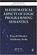Mathematical Aspects of Logic Programming Semantics

This book discusses applications of Logic Programming to computational logic and potential applications to the integration of models of computation, knowledge representation and reasoning, and the Semantic Web.

•Language, Proof and Logic (Jon Barwise, et al)

This book covers first-order language in a method appropriate for first and second courses in logic, and is specially useful to undergraduates of philosophy, computer science, mathematics, and linguistics.

•Logic, Programming and Prolog, 2nd Edition (Ulf Nilsson, et al)

This book introduces major new developments in a continually evolving field and includes such topics as concurrency and equational and constraint logic programming. What sets this book apart from others on logic programming is the breadth of its coverage.

•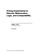Prolog Experiments in Discrete Math, Logic, and Computability

This book contains programming experiments that are designed to reinforce the learning of discrete mathematics, logic, and computability. The Prolog programming language is the tool used for the experiments in this book.

•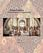Isomorphism - Mathematics of Programming (Xinyu Liu)

This book introduces the mathematics behind computer programming. It intents to tell: programming is isomorphic to mathematics. Just like in art and music, there are interesting stories and mathematicians behind the great minds.

Book Categories
 :All CategoriesTop Free BooksRecent BooksMiscellaneous BooksComputer EngineeringComputer LanguagesComputer ScienceData Science/DatabasesJava and Java EE (J2EE)Linux and UnixMathematicsMicrosoft and .NETMobile ComputingNetworking and CommunicationsSoftware EngineeringSpecial TopicsWeb Programming
Other Categories